# 1 Introduction

`PhosR` is a package for the comprehensive analysis of phosphoproteomic data. There are two major components to PhosR: processing and downstream analysis. PhosR consists of various processing tools for phosphoproteomic data including filtering, imputation, normalisaton and batch correction, enabling integration of multiple phosphoproteomic datasets. Downstream analytical tools consists of site- and protein-centric pathway analysis to evaluate activities of kinases and signalling pathways, large-scale kinase-substrate annotation from dynamic phosphoproteomic profiling, and visualisation and construction of signalomes present in the phosphoproteomic data of interest.

Below is a schematic overview of main componenets of PhosR, categorised into two broad steps of data analytics - processing and downstream analysis.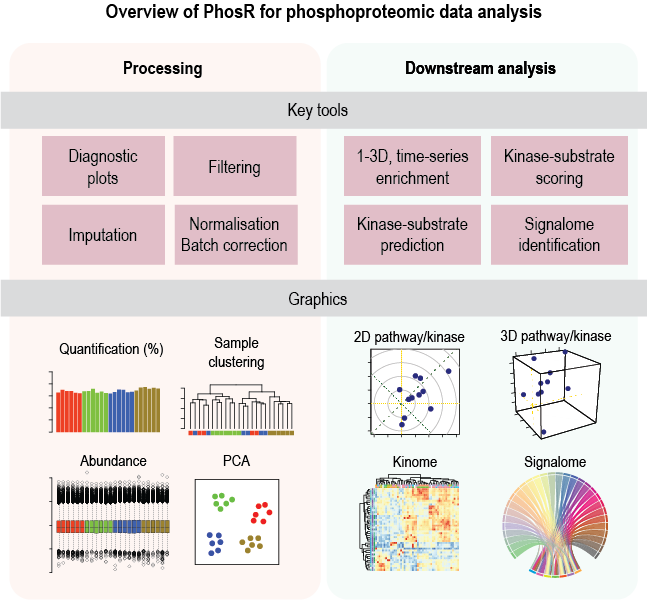Overview of PhosR methods

The purpose of this vignette is to illustrate some uses of `PhosR` and explain its key components.

# 2 Installation

Install the latest development version from GitHub using the `devtools` package:

``````library(devtools)
devtools::install_github("PYangLab/PhosR")``````

To install the Bioconductor version of PhosR, enter the following to your R console.

``````#if (!requireNamespace("BiocManager", quietly = TRUE))
#    install.packages("BiocManager")
#
#BiocManager::install("PhosR")``````

``````suppressPackageStartupMessages({
library(PhosR)
})``````

For demonstration purposes, we provide a rat L6 myotubes phosphoproteome dataset in our package. The data contains ratios of samples treated with AICAR, an analog of adenosine monophosphate that stimulates AMPK activity, insulin (Ins), or in combination (AICAR+Ins) with the basal condition. The raw data have been deposited to the ProteomeXchange Consortium via the PRIDE partner repository under PRIDE: PXD019127.

# 4 Setting up the PhosphoExperiment object

To increase compatibility of PhosR functions with diverse processed datasets, we have implemented a `PhosphoExperiment` (ppe) object based on the `SummarizedExperiment` class. To create the `PhosphoExperiment` object, you will need a quantification matrix where columns refer to cells and rows refer to sites.

``````data("phospho_L6_ratio")

ppe <- PhosphoExperiment(assays = list(Quantification = as.matrix(phospho.L6.ratio)))``````
``## Warning in .se_to_pe(se, UniprotID, GeneSymbol, Site, Residue, Sequence, : GeneSymbol is not specified. This may affect subsequent analysis steps.``
``## Warning in .se_to_pe(se, UniprotID, GeneSymbol, Site, Residue, Sequence, : Site is not specified. This may affect subsequent analysis steps.``
``## Warning in .se_to_pe(se, UniprotID, GeneSymbol, Site, Residue, Sequence, : Sequence is not specified. This may affect subsequent analysis steps.``
``## Warning in .se_to_pe(se, UniprotID, GeneSymbol, Site, Residue, Sequence, : Residue is not specified. This may affect subsequent analysis steps.``
``class(ppe)``
``````##  "PhosphoExperiment"
## attr(,"package")
##  "PhosR"``````

Additional annotation labels for the sites should be provided alongside the matrix. For each phosphosite, these include gene symbol, residue, position of phosphosite residue in the amino acid chain, and flanking sequence information of the phosphosite. Additional information such as the localisation probability may also be included.

``````GeneSymbol(ppe) <- sapply(strsplit(rownames(ppe), ";"), "[[", 2)
Residue(ppe) <- gsub("[0-9]","", sapply(strsplit(rownames(ppe), ";"), "[[", 3))
Site(ppe) <- as.numeric(gsub("[A-Z]","", sapply(strsplit(rownames(ppe), ";"), "[[", 3)))
Sequence(ppe) <- sapply(strsplit(rownames(ppe), ";"), "[[", 4)``````

Collectively, we can set up our data as following.

``````ppe <- PhosphoExperiment(assays = list(Quantification = as.matrix(phospho.L6.ratio)),
Site = as.numeric(gsub("[A-Z]","", sapply(strsplit(rownames(ppe), ";"), "[[", 3))),
GeneSymbol = sapply(strsplit(rownames(ppe), ";"), "[[", 2),
Residue = gsub("[0-9]","", sapply(strsplit(rownames(ppe), ";"), "[[", 3)),
Sequence = sapply(strsplit(rownames(ppe), ";"), "[[", 4))``````
``ppe``
``````## class: PhosphoExperiment
## dim: 6660 12
## assays(1): Quantification
## rownames(6660): Q6AYR1;TFG;S198;MSAFGLTDDQVSGPPSAPTEDRSGTPDSIAS
##   D3ZRN2;MED1;T1035;STGGSKSPGSSGRCQTPPGVATPPIPKITIQ ...
##   G3V7U4;LMNB1;S393;RKLLEGEEERLKLSPSPSSRVTVSRASSSRS
## rowData names(0):
## colnames(12): AICAR_exp1 AICAR_exp2 ... AICARIns_exp3 AICARIns_exp4
## colData names(0):``````

# 5 Part A. Preprocessing

## 5.1 Imputation

### 5.1.1 Introduction

`PhosR` is a package for the all-rounded analysis of phosphoproteomic data from processing to downstream analysis. This vignette will provide a step-by-step workflow of how PhosR can be used to process and analyse a a panel of phosphoproteomic datasets. As one of the first steps of data processing in phosphoproteomic analysis, we will begin by performing filtering and imputation of phosphoproteomic data with `PhosR`.

First, we will load the PhosR package. If you already haven’t done so, please install PhosR as instructed in the main page.

``````suppressPackageStartupMessages({
library(PhosR)
})``````

#### 5.1.2.1 Setting up the data

We assume that you will have the raw data processed using platforms frequently used for mass-spectrometry based proteomics such as MaxQuant. For demonstration purposes, we will take a parts of phosphoproteomic data generated by Humphrey et al. with accession number PXD001792. The dataset contains the phosphoproteomic quantifications of two mouse liver cell lines (Hepa1.6 and FL38B) that were treated with either PBS (mock) or insulin.

Let us load the PhosphoExperiment (ppe) object

``````data("phospho.cells.Ins.pe")

ppe <- phospho.cells.Ins.pe
class(ppe)``````
``````##  "PhosphoExperiment"
## attr(,"package")
##  "PhosR"``````

A quick glance of the object.

``ppe``
``````## class: PhosphoExperiment
## dim: 5000 24
## assays(1): Quantification
## rownames(5000): Q7TPV4;MYBBP1A;S1321;PQSALPKKRARLSLVSRSPSLLQSGVKKRRV
##   Q3UR85;MYRF;S304;PARAPSPPWPPQGPLSPGTGSLPLSIARAQT ...
##   P28659-4;NA;S18;AFKLDFLPEMMVDHCSLNSSPVSKKMNGTLD
##   E9Q8I9;FRY;S1380;HNIELVDSRLLLPGSSPSSPEDEVKDREGEV
## rowData names(0):
## colnames(24): Intensity.FL83B_Control_1 Intensity.FL83B_Control_2 ...
##   Intensity.Hepa1.6_Ins_5 Intensity.Hepa1.6_Ins_6
## colData names(0):``````

We will take the grouping information from `colnames` of our matrix.

``grps = gsub("_[0-9]{1}", "", colnames(ppe))``

For each cell line, there are two conditions (Control vs Insulin-stimulated) and 6 replicates for each condition.

``````# FL38B
gsub("Intensity.", "", grps)[1:12]``````
``````##   "FL83B_Control" "FL83B_Control" "FL83B_Control" "FL83B_Control"
##   "FL83B_Control" "FL83B_Control" "FL83B_Ins"     "FL83B_Ins"
##   "FL83B_Ins"     "FL83B_Ins"     "FL83B_Ins"     "FL83B_Ins"``````
``````# Hepa1
gsub("Intensity.", "", grps)[13:24]``````
``````##   "Hepa1.6_Control" "Hepa1.6_Control" "Hepa1.6_Control" "Hepa1.6_Control"
##   "Hepa1.6_Control" "Hepa1.6_Control" "Hepa1.6_Ins"     "Hepa1.6_Ins"
##   "Hepa1.6_Ins"     "Hepa1.6_Ins"     "Hepa1.6_Ins"     "Hepa1.6_Ins"``````

Note that there are in total 24 samples and 5,000 phosphosites profiled.

``dim(ppe)``
``##  5000   24``

### 5.1.3 Filtering of phosphosites

Next, we will perform some filtering of phosphosites so that only phosphosites with quantification for at least 50% of the replicates in at least one of the conditions are retained. For this filtering step, we use the `selectGrps` function. The filtering leaves us with 1,772 phosphosites.

``````ppe_filtered <- selectGrps(ppe, grps, 0.5, n=1)
dim(ppe_filtered)``````
``##  1772   24``

`selectGrps` gives you the option to relax the threshold for filtering. The filtering threshold can therefore be optimized for each dataset.

``````# In cases where you have fewer replicates ( e.g.,triplicates), you may want to
# select phosphosites quantified in 70% of replicates.
ppe_filtered_v1 <- selectGrps(ppe, grps, 0.7, n=1)
dim(ppe_filtered_v1)``````
``##  1330   24``

### 5.1.4 Imputation of phosphosites

We can proceed to imputation now that we have filtered for suboptimal phosphosites. To take advantage of data structure and experimental design, PhosR provides users with a lot of flexibility for imputation. There are three functions for imputation: `scImpute`,`tInmpute`, and `ptImpute`. Here, we will demonstrate the use of `scImpute` and `ptImpute`.

#### 5.1.4.1 Site- and condition-specific imputation

The `scImpute` function is used for site- and condition-specific imputation. A pre-defined thereshold is used to select phosphosites to impute. Phosphosites with missing values equal to or greater than a predefined value will be imputed by sampling from the empirical normal distribution constructed from the quantification values of phosphosites from the same condition.

``````set.seed(123)
ppe_imputed_tmp <- scImpute(ppe_filtered, 0.5, grps)[,colnames(ppe_filtered)]``````

In the above example, only phosphosites that are quantified in more than 50% of samples from the same condition will be imputed.

#### 5.1.4.2 Paired tail-based imputation

We then perform paired tail-based imputation on the dataset imputed with `scImpute`. Paired tail-based imputation performs imputation of phosphosites that have missing values in all replicates in one condition (e.g. in basal) but not in another condition (e.g., in stimulation). This method of imputation ensures that we do not accidentally filter phosphosites that seemingly have low detection rate.

As for `scImpute`, we can set a predefined threshold to in another condition (e.g. stimulation), the tail-based imputation is applied to impute for the missing values in the first condition.

As for `scImpute`, we can set a predefined threshold to in another condition (e.g. stimulation), the tail-based imputation is applied to impute for the missing values in the first condition.

``````set.seed(123)
ppe_imputed <- ppe_imputed_tmp
ppe_imputed[,seq(6)] <- ptImpute(ppe_imputed[,seq(7,12)],
ppe_imputed[,seq(6)],
percent1 = 0.6, percent2 = 0, paired = FALSE)``````
``## idx1: 12``
``````ppe_imputed[,seq(13,18)] <- ptImpute(ppe_imputed[,seq(19,24)],
ppe_imputed[,seq(13,18)],
percent1 = 0.6, percent2 = 0,
paired = FALSE)``````
``## idx1: 29``

Lastly, we perform normalisation of the filtered and imputed phosphoproteomic data.

``ppe_imputed_scaled <- medianScaling(ppe_imputed, scale = FALSE, assay = "imputed")``

### 5.1.5 Quantification plots

A useful function in `PhosR` is to visualize the percentage of quantified sites before and after filtering and imputation. The main inputs of `plotQC` are the quantification matrix, sample labels (equating the column names of the matrix), an integer indicating the panel to plot, and lastly, a color vector. To visualize the percentage of quantified sites, use the `plotQC` function and set panel = “quantify” to visualise bar plots of samples.

``````p1 = plotQC(SummarizedExperiment::assay(ppe_filtered,"Quantification"),
labels=colnames(ppe_filtered),
panel = "quantify", grps = grps)
p2 = plotQC(SummarizedExperiment::assay(ppe_imputed_scaled,"scaled"),
labels=colnames(ppe_imputed_scaled), panel = "quantify", grps = grps)
ggpubr::ggarrange(p1, p2, nrow = 1)``````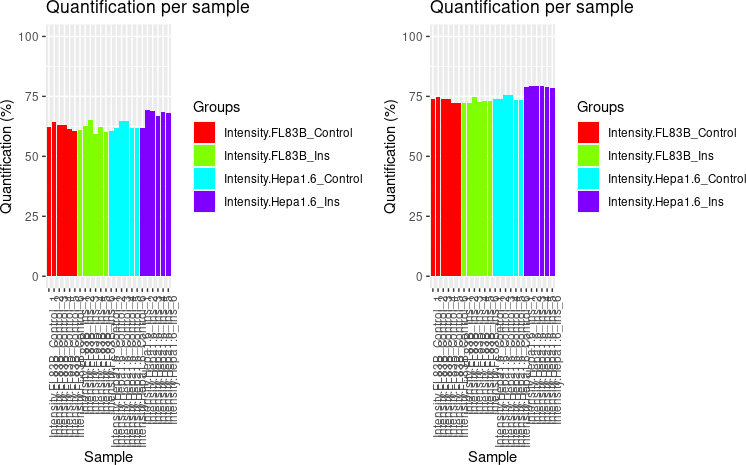By setting panel = “dendrogram”, we can visualise the results of unsupervised hierarchical clustering of samples as a dendrogram. The dendrogram demonstrates that imputation has improved the clustering of the samples so that replicates from the same conditions cluster together.

``````p1 = plotQC(SummarizedExperiment::assay(ppe_filtered,"Quantification"),
labels=colnames(ppe_filtered), panel = "dendrogram",
grps = grps)
p2 = plotQC(SummarizedExperiment::assay(ppe_imputed_scaled,"scaled"),
labels=colnames(ppe_imputed_scaled),
panel = "dendrogram", grps = grps)
ggpubr::ggarrange(p1, p2, nrow = 1)``````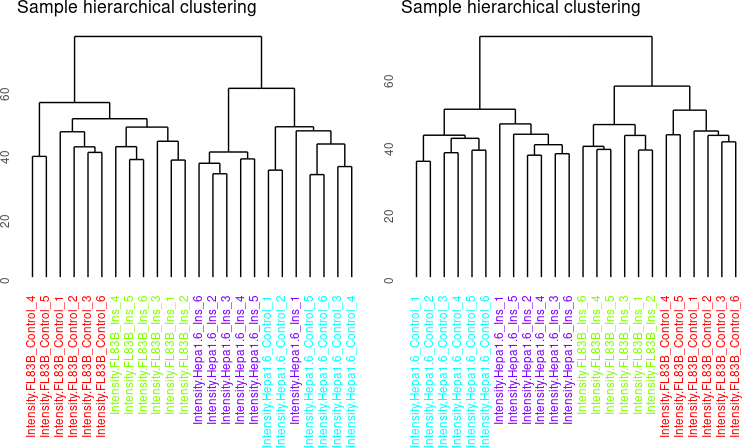We can now move onto the next step in the `PhosR` workflow: integration of datasets and batch correction.

## 5.2 Batch correction

### 5.2.1 Introduction

A common but largely unaddressed challenge in phosphoproteomic data analysis is to correct for batch effect. Without correcting for batch effect, it is often not possible to analyze datasets in an integrative manner. To perform data integration and batch effect correction, we identified a set of stably phosphorylated sites (SPSs) across a panel of phosphoproteomic datasets and, using these SPSs, implemented a wrapper function of RUV-III from the `ruv` package called `RUVphospho`.

Note that when the input data contains missing values, imputation should be performed before batch correction since RUV-III requires a complete data matrix. The imputed values are removed by default after normalisation but can be retained for downstream analysis if the users wish to use the imputed matrix. This vignette will provide an example of how PhosR can be used for batch correction.

``````suppressPackageStartupMessages({
library(PhosR)
library(stringr)
library(ggplot2)
})``````

In this example, we will use L6 myotube phosphoproteome dataset (with accession number PXD019127) and the SPSs we identified from a panel of phosphoproteomic datasets (please refer to our preprint for the full list of the datasets used). The `SPSs` will be used as our `negative control` in RUV normalisation.

``````data("phospho_L6_ratio_pe")
data("SPSs")

ppe <- phospho.L6.ratio.pe
ppe``````
``````## class: PhosphoExperiment
## dim: 6654 12
## assays(1): Quantification
## rownames(6654): D3ZNS8;AAAS;S495;THIPLYFVNAQFPRFSPVLGRAQEPPAGGGG
##   Q9R0Z7;AAGAB;S210;RSVGSAESCQCEQEPSPTAERTESLPGHRSG ...
##   D3ZG78;ZZEF1;S1516;SGPSAAEVSTAEEPSSPSTPTRRPPFTRGRL
##   D3ZG78;ZZEF1;S1535;PTRRPPFTRGRLRLLSFRSMEETRPVPTVKE
## rowData names(0):
## colnames(12): AICAR_exp1 AICAR_exp2 ... AICARIns_exp3 AICARIns_exp4
## colData names(0):``````

### 5.2.3 Setting up the data

The L6 myotube data contains phosphoproteomic samples from three treatment conditions each with quadruplicates. Myotube cells were treated with either AICAR or Insulin (Ins), which are both important modulators of the insulin signalling pathway, or both (AICARIns) before phosphoproteomic analysis.

``colnames(ppe)[grepl("AICAR_", colnames(ppe))]``
``##  "AICAR_exp1" "AICAR_exp2" "AICAR_exp3" "AICAR_exp4"``
``colnames(ppe)[grepl("^Ins_", colnames(ppe))]``
``##  "Ins_exp1" "Ins_exp2" "Ins_exp3" "Ins_exp4"``
``colnames(ppe)[grepl("AICARIns_", colnames(ppe))]``
``##  "AICARIns_exp1" "AICARIns_exp2" "AICARIns_exp3" "AICARIns_exp4"``

Note that we have in total 6654 quantified phosphosites and 12 samples in total.

``dim(ppe)``
``##  6654   12``

We have already performed the relevant processing steps to generate a dense matrix. Please refer to the `imputation` page to perform filtering and imputation of phosphosites in order to generate a matrix without any missing values.

``sum(is.na(SummarizedExperiment::assay(ppe,"Quantification")))``
``##  0``

We will extract phosphosite labels.

``````sites = paste(sapply(GeneSymbol(ppe), function(x)x),";",
sapply(Residue(ppe), function(x)x),
sapply(Site(ppe), function(x)x),
";", sep = "")``````

Lastly, we will take the grouping information from `colnames` of our matrix.

``````# take the grouping information
grps = gsub("_.+", "", colnames(ppe))
grps``````
``````##   "AICAR"    "AICAR"    "AICAR"    "AICAR"    "Ins"      "Ins"
##   "Ins"      "Ins"      "AICARIns" "AICARIns" "AICARIns" "AICARIns"``````

### 5.2.4 Diagnosing batch effect

There are a number of ways to diagnose batch effect. In `PhosR`, we make use of two visualisation methods to detect batch effect: dendrogram of hierarchical clustering and a principal component analysis (PCA) plot. We use the `plotQC` function we introduced in the imputation section of the vignette.

By setting panel = “dendrogram”, we can plot the dendrogram illustrating the results of unsupervised hierarchical clustering of our 12 samples. Clustering results of the samples demonstrate that there is a strong batch effect (where batch denoted as expX, where X refers to the batch number). This is particularly evident for samples from Ins and AICARIns treated conditions.

``````plotQC(SummarizedExperiment::assay(ppe,"Quantification"), panel = "dendrogram",
grps=grps, labels = colnames(ppe)) +
ggplot2::ggtitle("Before batch correction")``````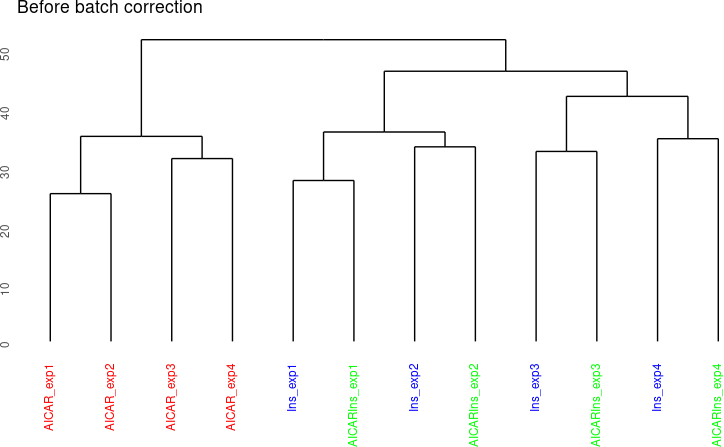We can also visualise the samples in PCA space by setting panel = “pca”. The PCA plot demonstrates aggregation of samples by batch rather than treatment groups (each point represents a sample coloured by treatment condition). It has become clearer that even within the AICAR treated samples, there is some degree of batch effect as data points are separated between samples from batches 1 and 2 and those from batches 3 and 4.

``````plotQC(SummarizedExperiment::assay(ppe,"Quantification"), grps=grps,
labels = colnames(ppe), panel = "pca") +
ggplot2::ggtitle("Before batch correction")``````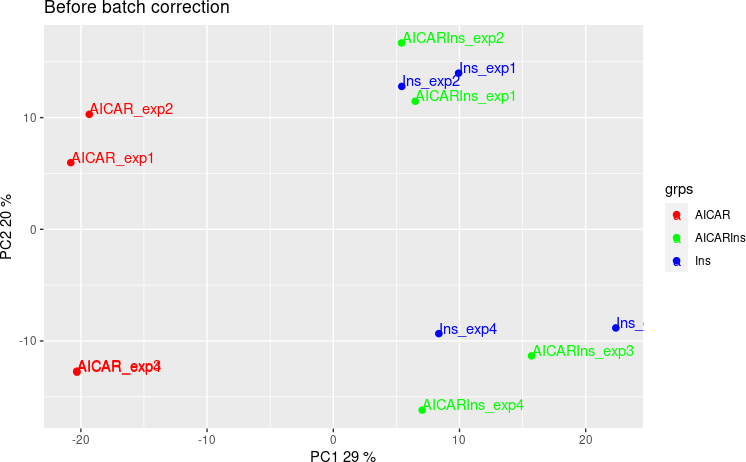### 5.2.5 Correcting batch effect

We have now diagnosed that our dataset exhibits batch effect that is driven by experiment runs for samples treated with three different conditions. To address this batch effect, we correct for this unwanted variation in the data by utilising our pre-defined SPSs as a negative control for `RUVphospho`.

First, we construct a design matrix by condition.

``````design = model.matrix(~ grps - 1)
design``````
``````##    grpsAICAR grpsAICARIns grpsIns
## 1          1            0       0
## 2          1            0       0
## 3          1            0       0
## 4          1            0       0
## 5          0            0       1
## 6          0            0       1
## 7          0            0       1
## 8          0            0       1
## 9          0            1       0
## 10         0            1       0
## 11         0            1       0
## 12         0            1       0
## attr(,"assign")
##  1 1 1
## attr(,"contrasts")
## attr(,"contrasts")\$grps
##  "contr.treatment"``````

We will then use the `RUVphospho` function to normalise the data. Besides the quantification matrix and the design matrix, there are two other important inputs to `RUVphospho`: 1) the ctl argument is an integer vector denoting the position of SPSs within the quantification matrix 2) k parameter is an integer denoting the expected number of experimental (e.g., treatment) groups within the data

``````# phosphoproteomics data normalisation and batch correction using RUV
ctl = which(sites %in% SPSs)
ppe = RUVphospho(ppe, M = design, k = 3, ctl = ctl)``````

### 5.2.6 Quality control

As quality control, we will demonstrate and evaluate our normalisation method with hierarchical clustering and PCA plot using again `plotQC`. Both the hierarchical clustering and PCA results demonstrate the normalisation procedure in `PhosR` facilitates effective batch correction.

``````# plot after batch correction
p1 = plotQC(SummarizedExperiment::assay(ppe, "Quantification"), grps=grps,
labels = colnames(ppe), panel = "dendrogram" )
p2 = plotQC(SummarizedExperiment::assay(ppe, "normalised"), grps=grps,
labels = colnames(ppe), panel="dendrogram")
ggpubr::ggarrange(p1, p2, nrow = 1)``````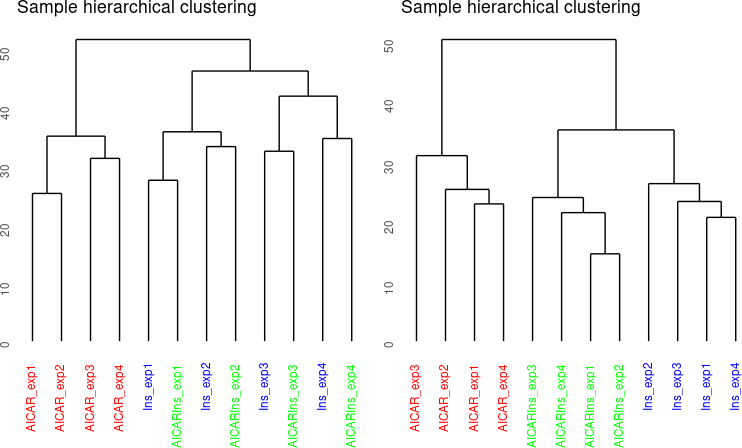``````# plot after batch correction
p1 = plotQC(SummarizedExperiment::assay(ppe, "Quantification"), panel = "pca",
grps=grps, labels = colnames(ppe)) +
ggplot2::ggtitle("Before Batch correction")
p2 = plotQC(SummarizedExperiment::assay(ppe, "normalised"), grps=grps,
labels = colnames(ppe), panel="pca") +
ggplot2::ggtitle("After Batch correction")
ggpubr::ggarrange(p1, p2, nrow = 2)``````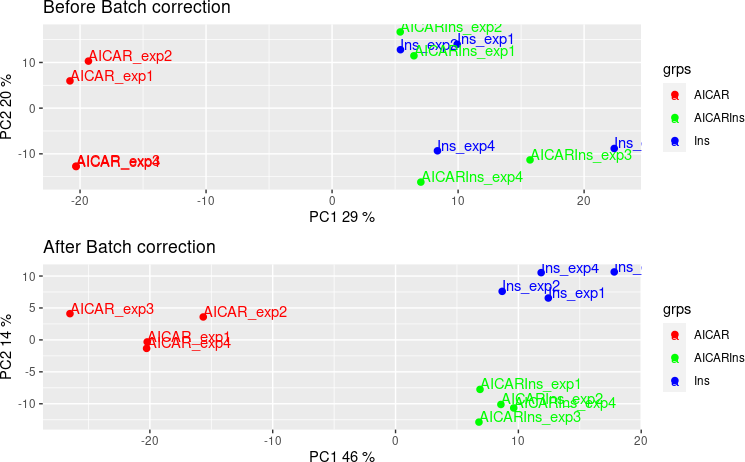### 5.2.7 Generating SPSs

We note that the current SPS are derived from phosphoproteomic datasets derived from mouse cells. To enable users working on phosphoproteomic datasets derived from other species, we have developed a function `getSPS` that takes in multiple phosphoproteomics datasets stored as phosphoExperiment objects to generate set of SPS. Users can use their in-house or public datasets derived from the same speciies to generate species-specific SPS, which they can use to normalise their data.Below demonstrate the usage of the `getSPS` function.

First, load datasets to use for SPS generation.

``````data("phospho_L6_ratio_pe")
data("phospho.liver.Ins.TC.ratio.RUV.pe")
data("phospho.cells.Ins.pe")
ppe1 <- phospho.L6.ratio.pe
ppe2 <- phospho.liver.Ins.TC.ratio.RUV.pe
# ppe2 <- phospho.L1.Ins.ratio.subset.pe
ppe3 <- phospho.cells.Ins.pe``````

Filter, impute, and transform ppe3 (other inputs have already been processed).

``````grp3 = gsub('_[0-9]{1}', '', colnames(ppe3))
ppe3 <- selectGrps(ppe3, grps = grp3, 0.5, n=1)
ppe3 <- tImpute(ppe3)
FL83B.ratio <- SummarizedExperiment::assay(ppe3, "imputed")[, 1:12] -
rowMeans(SummarizedExperiment::assay(ppe3, "imputed")[,grep("FL83B_Control",
colnames(ppe3))])
Hepa.ratio <- SummarizedExperiment::assay(ppe3,"imputed")[, 13:24] -
rowMeans(SummarizedExperiment::assay(ppe3,"imputed")[,grep("Hepa1.6_Control",
colnames(ppe3))])
SummarizedExperiment::assay(ppe3, "Quantification") <-
cbind(FL83B.ratio, Hepa.ratio)``````

Generate inputs of `getSPS`.

``````ppe.list <- list(ppe1, ppe2, ppe3)
# ppe.list <- list(ppe1, ppe3)

cond.list <- list(grp1 = gsub("_.+", "", colnames(ppe1)),
grp2 = str_sub(colnames(ppe2), end=-5),
grp3 = str_sub(colnames(ppe3), end=-3))``````

Finally run `getSPS` to generate list of SPSs.

``inhouse_SPSs <- getSPS(ppe.list, conds = cond.list)``
``## Warning: there aren't enough overlappling sites``
``head(inhouse_SPSs)``
``````##  "TMPO;S67;"    "RBM5;S621;"   "SRRM1;S427;"  "THOC2;S1417;" "SSB;S92;"
##  "PPIG;S252;"``````

Run `RUVphospho` using the newly generateed SPSs. (NOTE that we do not expect the results to be reproducible as the exempler SPSs have been generated from dummy datasets that have been heavily filtered.)

``````sites = paste(sapply(GeneSymbol(ppe), function(x)x),";",
sapply(Residue(ppe), function(x)x),
sapply(Site(ppe), function(x)x), ";", sep = "")

ctl = which(sites %in% inhouse_SPSs)
ppe = RUVphospho(ppe, M = design, k = 3, ctl = ctl)``````

# 6 Part B. Downstream analysis

## 6.1 Pathway analysis

### 6.1.1 Introduction

Most phosphoproteomic studies have adopted a phosphosite-level analysis of the data. To enable phosphoproteomic data analysis at the gene level, `PhosR` implements both site- and gene-centric analyses for detecting changes in kinase activities and signalling pathways through traditional enrichment analyses (over-representation or rank-based gene set test, together referred to as‘1-dimensiona